Parameter Passing Giuseppe Attardi Universit di Pisa Parameter

• Slides: 37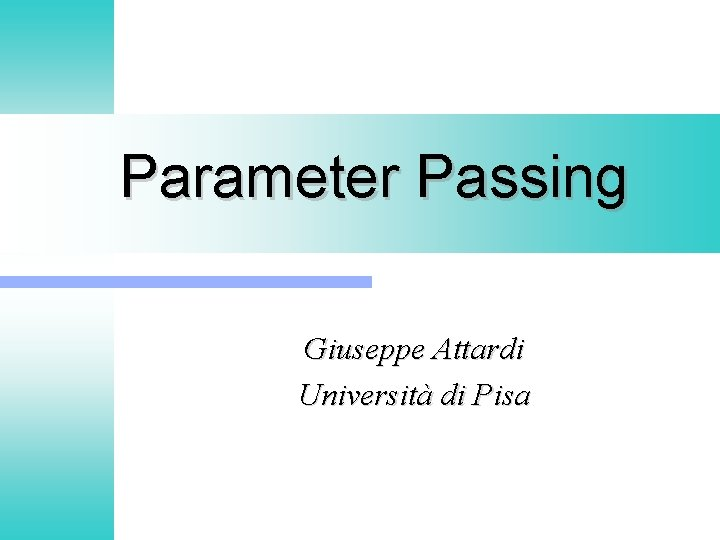Parameter Passing Giuseppe Attardi Università di Pisa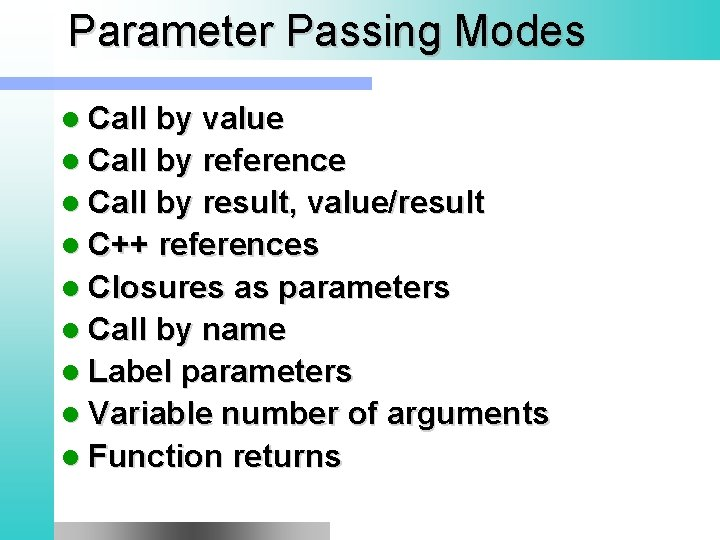Parameter Passing Modes l Call by value l Call by reference l Call by result, value/result l C++ references l Closures as parameters l Call by name l Label parameters l Variable number of arguments l Function returns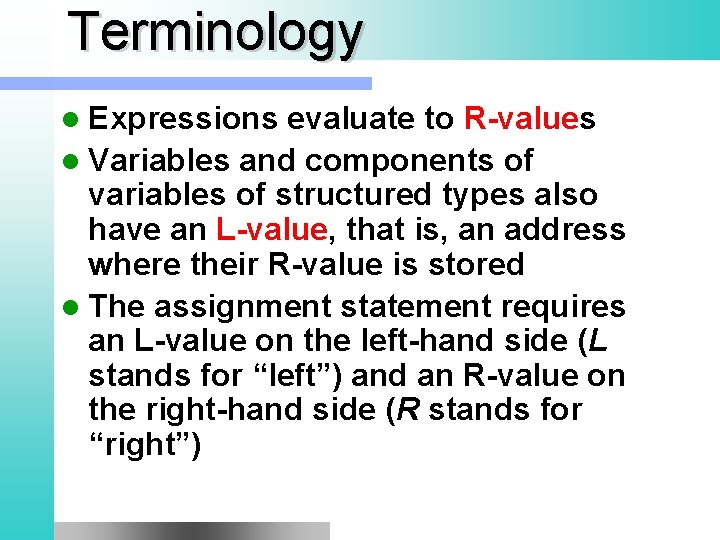Terminology l Expressions evaluate to R-values l Variables and components of variables of structured types also have an L-value, that is, an address where their R-value is stored l The assignment statement requires an L-value on the left-hand side (L stands for “left”) and an R-value on the right-hand side (R stands for “right”)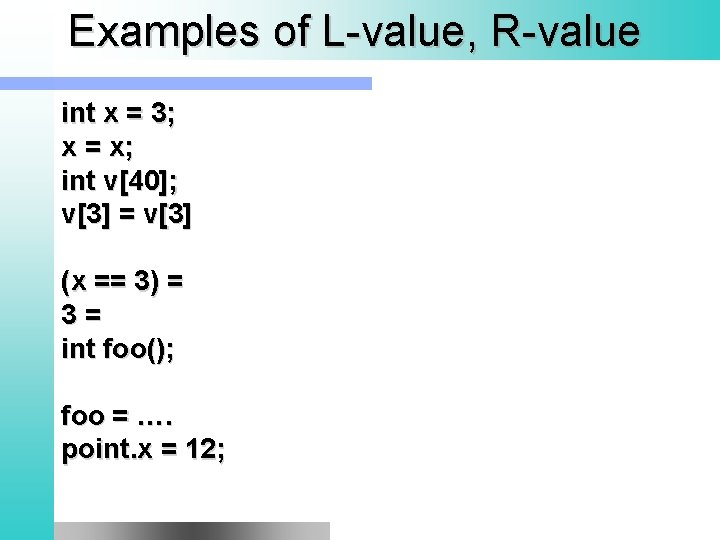Examples of L-value, R-value int x = 3; x = x; int v; v = v (x == 3) = 3= int foo(); foo = …. point. x = 12;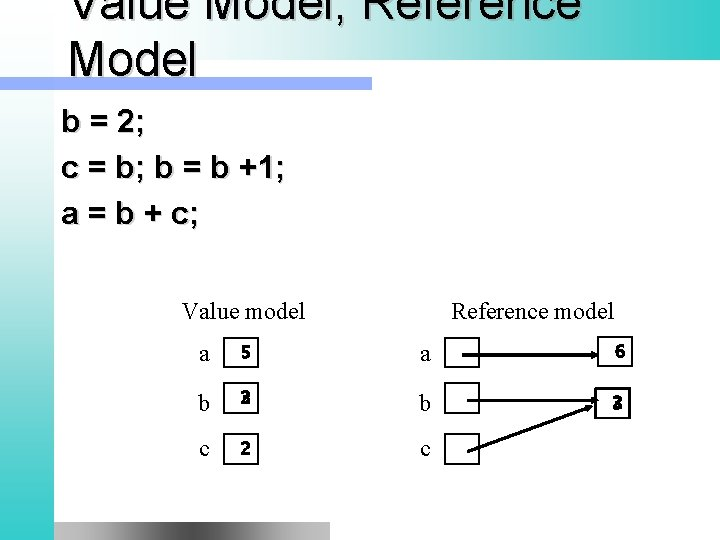Value Model, Reference Model b = 2; c = b; b = b +1; a = b + c; Value model Reference model a 5 a 6 b 3 2 b 2 3 c 2 cExample class Point { int x, y; } Point p = Point(3, 4); Point p 2 = p; p. x = 5; p 2. x ? foo(p); int i = 3; Integer i = new Integer(3);C Language void foo (int* x) { *x = 3; } struct Point { int x, y}; Point p; bar(&p); void bar(Point* p) { p->x = 3; } Point* p = new Point(); p->x = 5; Point* p 2 = p; bar(p 2);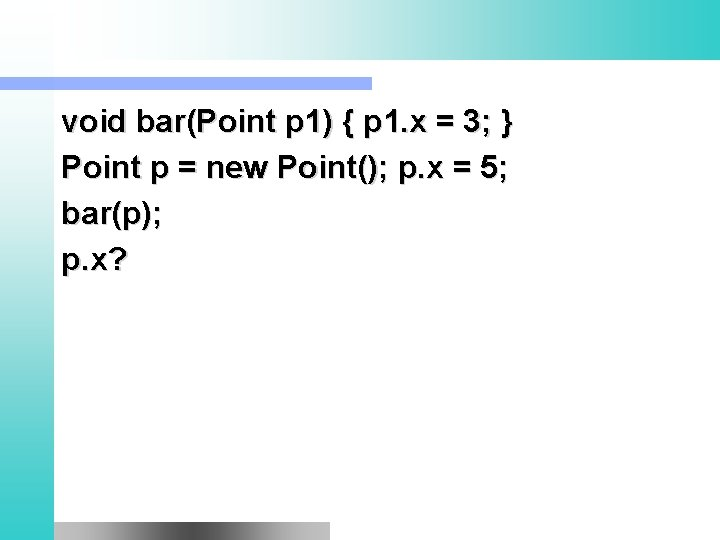void bar(Point p 1) { p 1. x = 3; } Point p = new Point(); p. x = 5; bar(p); p. x?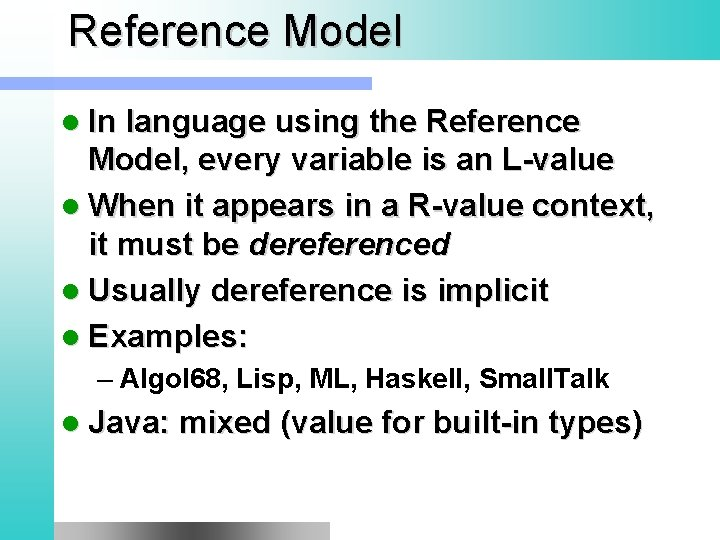Reference Model l In language using the Reference Model, every variable is an L-value l When it appears in a R-value context, it must be dereferenced l Usually dereference is implicit l Examples: – Algol 68, Lisp, ML, Haskell, Small. Talk l Java: mixed (value for built-in types)Call by value l The value of the R-value of the actual parameter is copied into the formal parameter at invocation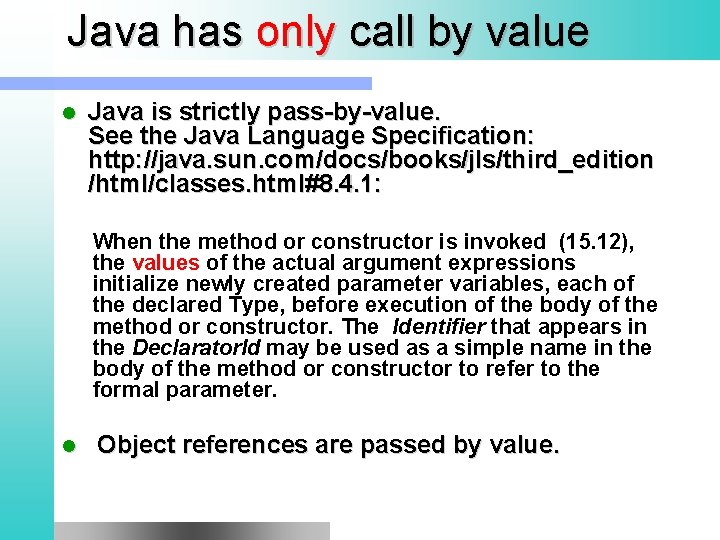Java has only call by value l Java is strictly pass-by-value. See the Java Language Specification: http: //java. sun. com/docs/books/jls/third_edition /html/classes. html#8. 4. 1: When the method or constructor is invoked (15. 12), the values of the actual argument expressions initialize newly created parameter variables, each of the declared Type, before execution of the body of the method or constructor. The Identifier that appears in the Declarator. Id may be used as a simple name in the body of the method or constructor to refer to the formal parameter. l Object references are passed by value.Call by result l The value of the formal parameter is copied into the actual parameter (which must have an L-value) at procedure return.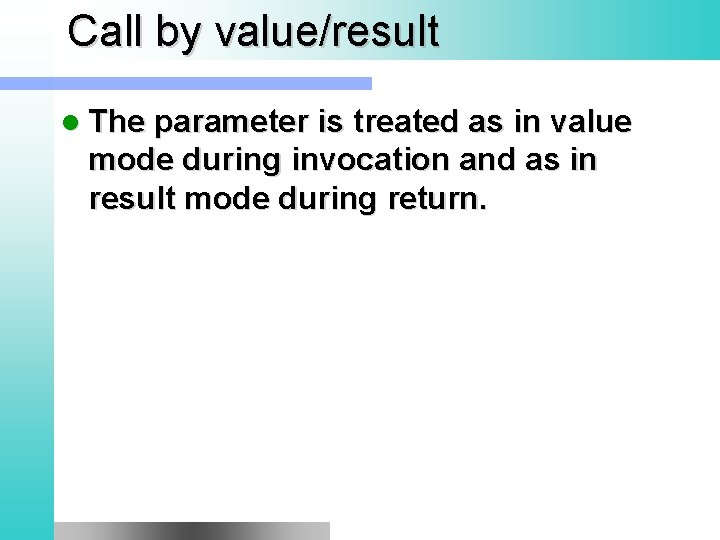Call by value/result l The parameter is treated as in value mode during invocation and as in result mode during return.Note foo (inout x) { … x …} foo 1 (ref x) { … x = x + 1; … } foo 1(v); s = “asdasd”; foo 1(s); // OK foo 1(“asdasd”); // KO foo 1(3); // KO int y = 7; foo 1(y); y?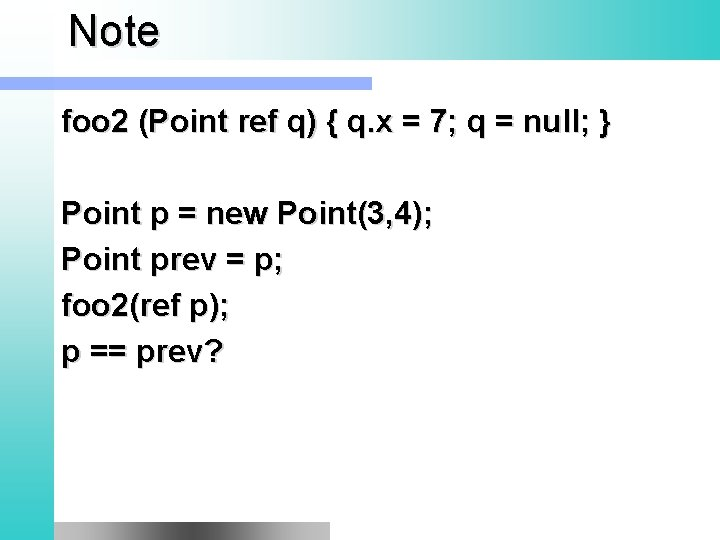Note foo 2 (Point ref q) { q. x = 7; q = null; } Point p = new Point(3, 4); Point prev = p; foo 2(ref p); p == prev?Note void foo (ref int x) { x = x + 3; x = x/0; } int z = 2; foo(z);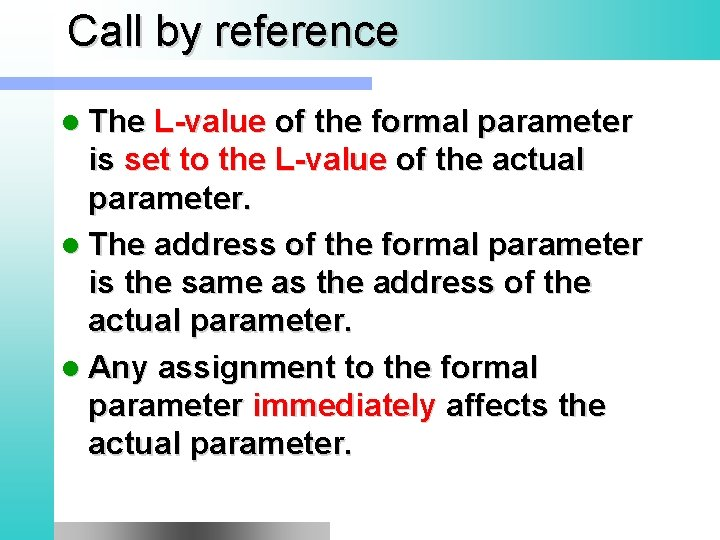Call by reference l The L-value of the formal parameter is set to the L-value of the actual parameter. l The address of the formal parameter is the same as the address of the actual parameter. l Any assignment to the formal parameter immediately affects the actual parameter.Note // C# void foo(int ref x) { x += 1; } // C++ void foo(int& x) { x += 1; } int a; a = 0; foo(ref a); // C# foo(a); // C++ foo(p);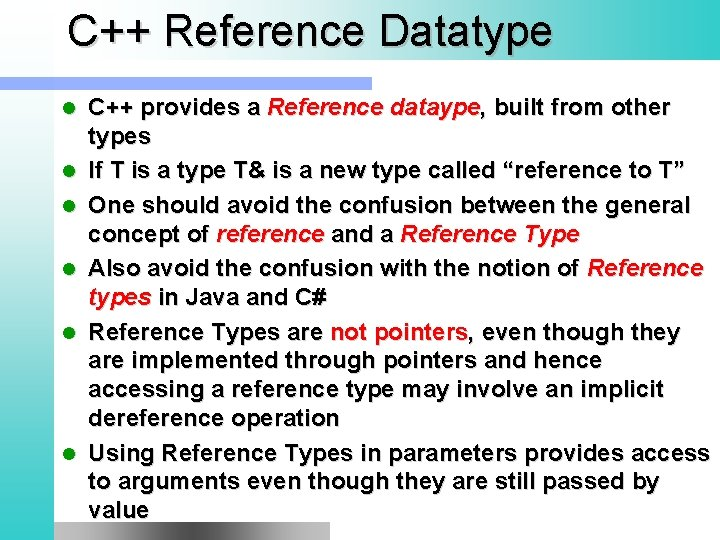C++ Reference Datatype l l l C++ provides a Reference dataype, built from other types If T is a type T& is a new type called “reference to T” One should avoid the confusion between the general concept of reference and a Reference Type Also avoid the confusion with the notion of Reference types in Java and C# Reference Types are not pointers, even though they are implemented through pointers and hence accessing a reference type may involve an implicit dereference operation Using Reference Types in parameters provides access to arguments even though they are still passed by value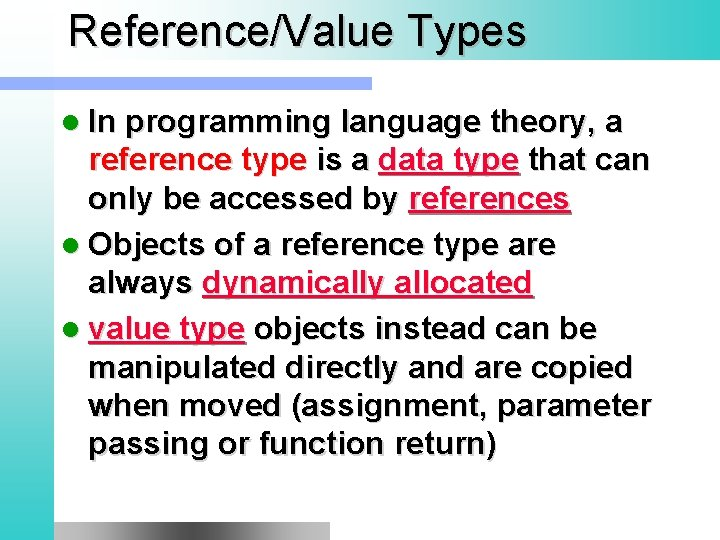Reference/Value Types l In programming language theory, a reference type is a data type that can only be accessed by references l Objects of a reference type are always dynamically allocated l value type objects instead can be manipulated directly and are copied when moved (assignment, parameter passing or function return)C++ Reference Type Example void foo(int& x) { int& z = x; x = x + 1; } int y = 7; foo(y); y? foo(3); // only feasible if x was // declared as const int&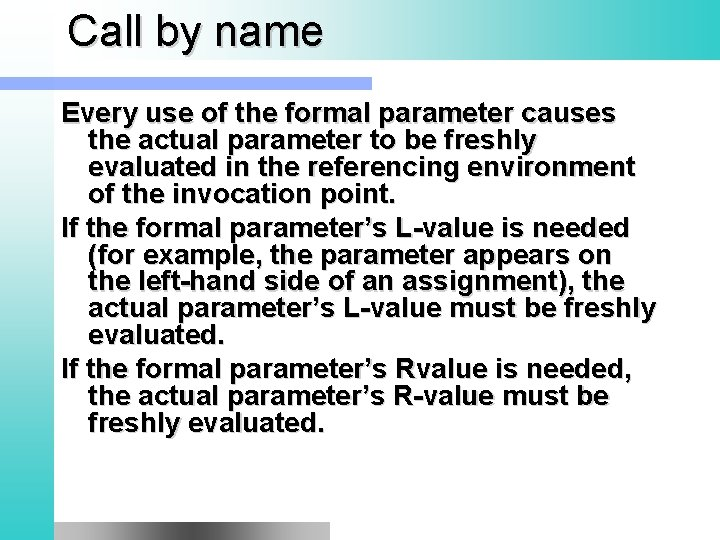Call by name Every use of the formal parameter causes the actual parameter to be freshly evaluated in the referencing environment of the invocation point. If the formal parameter’s L-value is needed (for example, the parameter appears on the left-hand side of an assignment), the actual parameter’s L-value must be freshly evaluated. If the formal parameter’s Rvalue is needed, the actual parameter’s R-value must be freshly evaluated.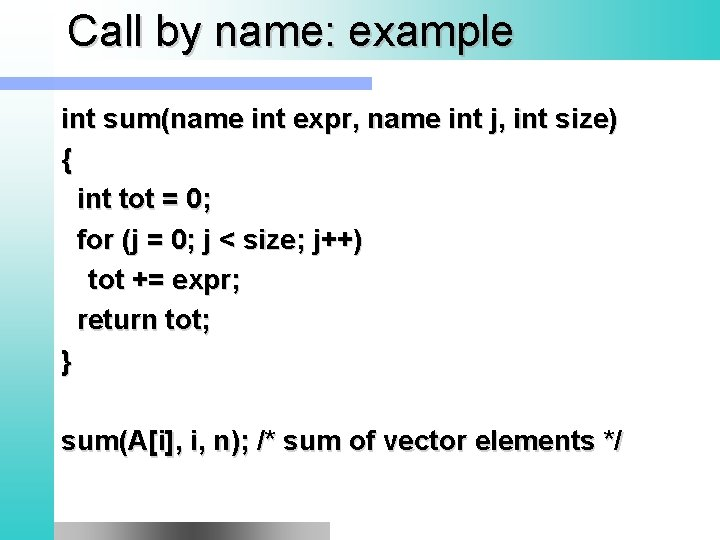Call by name: example int sum(name int expr, name int j, int size) { int tot = 0; for (j = 0; j < size; j++) tot += expr; return tot; } sum(A[i], i, n); /* sum of vector elements */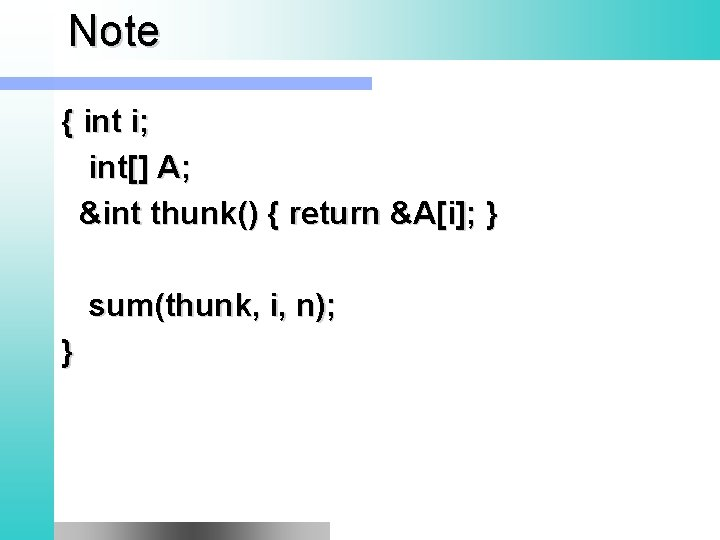Note { int i; int[] A; &int thunk() { return &A[i]; } sum(thunk, i, n); }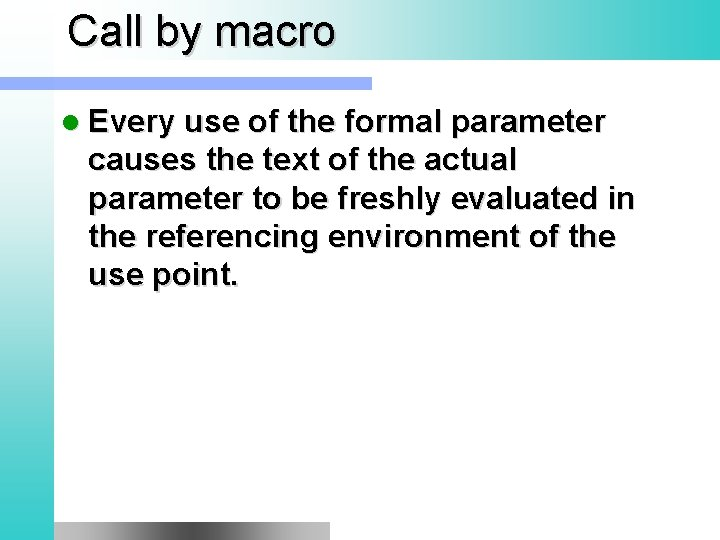Call by macro l Every use of the formal parameter causes the text of the actual parameter to be freshly evaluated in the referencing environment of the use point.Macro Example #DEFINE foo(x) x + x foo(read()) read() + read() inline int foo(int x) { return x + x; } int foo(int& x, int &y) { x++; y++; return x + y; } Foo(a, a);Parameter passing modes Implementation Operations Change to actual? Alias? Value RW No no In, const Value, reference RO No Maybe Out (C#, Ada) Value, reference WO Yes Maybe Value/result Value RW Yes No Var, ref Reference RW Yes Sharing Value, reference RW Yes Maybe In out Value, reference RW Yes Maybe Name Closure RW Yes yesExercise l swap(x, y) using just call-by-value void swap(int x, int y) { int temp; temp = x; x = y; y = temp; }; Does not work.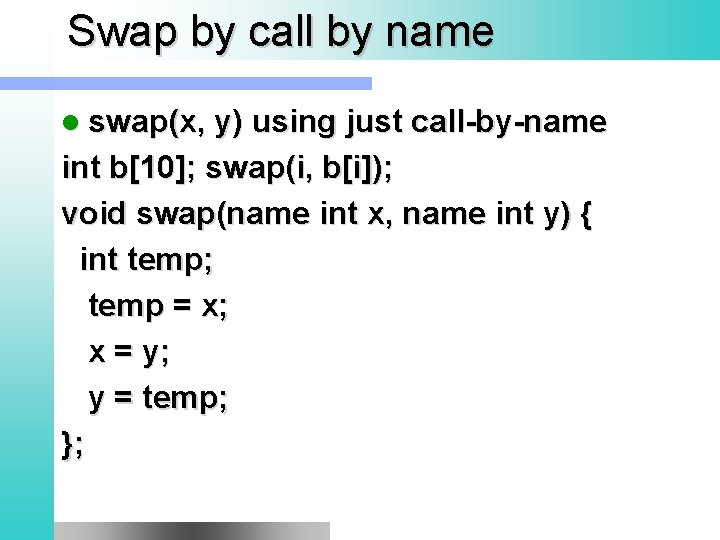Swap by call by name l swap(x, y) using just call-by-name int b; swap(i, b[i]); void swap(name int x, name int y) { int temp; temp = x; x = y; y = temp; };Swap with C# ref void swap(int ref x, int ref y) { int tmp = y; y = x; x = tmp; } int a = 3, b =5; swap(a, b);Note on List l = new List(); Student s = new Student(); l. add(s); float pi = 3. 14; l. add(pi); (new Integer(314)). to. String(); s. to. String();Note int a[] = new int; int b[] = new int; swap(a, b); a swap(i, a[i]); int temp; temp = x; x = y; y = temp; a=3, b=5; a=5, b=3; void swap(inout int x, inout int y) { int temp; temp = x; x = y; y = temp; };Exercise l swap(x, y) using just call-by-name swap(name x, name y) => { } Does it work?Call by name Counter example: swap(i, A[i]) (i=3, A = 4) => (i=4, A = 4, A unchanged)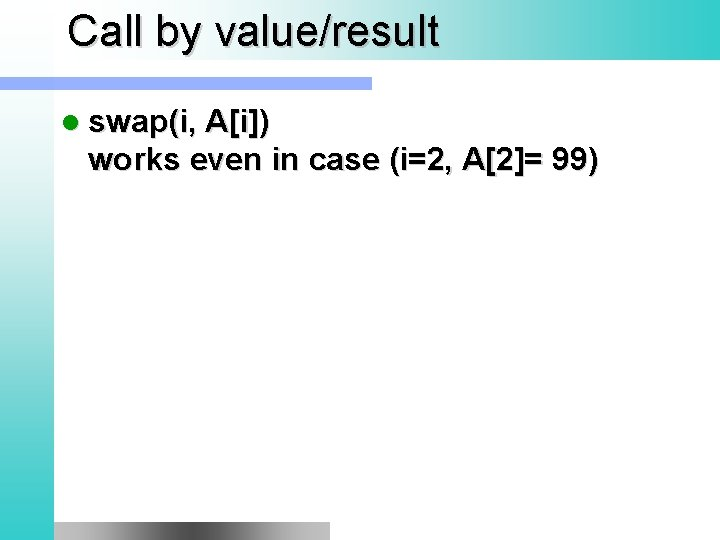Call by value/result l swap(i, A[i]) works even in case (i=2, A= 99)Call by value l l Copies argument Special cases: – array – struct typedef struct { int x, y} Pair; Pair q; zed(q); Pair foo() { Pair p; return p; } Pair p = foo(); stack. push_back(Pair(2, 3)); int  v; bar(v); std: : string s; bad(s);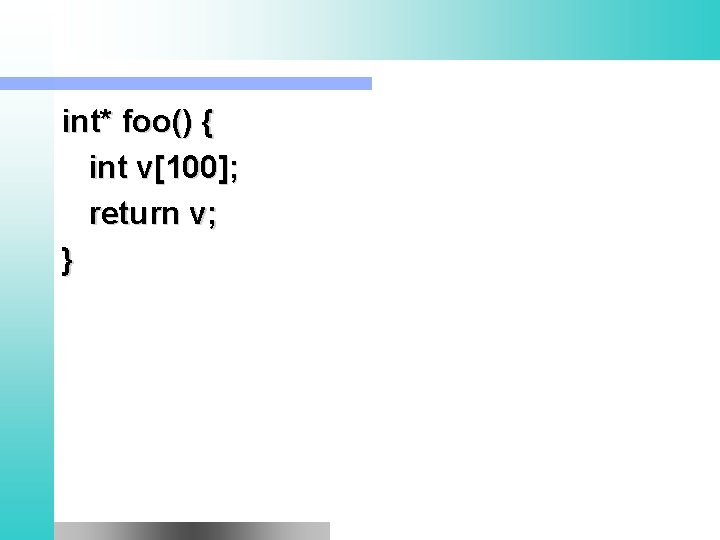int* foo() { int v; return v; }